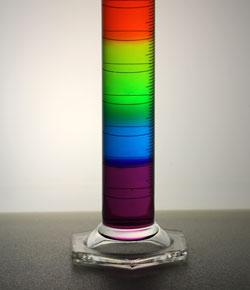# Moore 1.3 The International System Of Units (SI)

6 Questions | Attempts: 190
ShareSettingsMoore section 1. 3 reading check questions

• 1.
1 dm is equivalent to
• A.

0.1 m

• B.

0.1 cm

• C.

0.1 km

• D.

0.1 mm

• 2.
1 dm^3 measures
• A.

Length

• B.

Surface area

• C.

Volume

• D.

Density

• 3.
1 dm^3 is equivalent to
• A.

0.001 cm^3

• B.

0.001 m^3

• C.

0.001 km^3

• D.

0.001 mm^3

• 4.
Greek symbol used for density is
• A.

υ

• B.

π

• C.

ρ

• D.

θ

• 5.
Which of the following is the unit for density?
• A.

G/cm^3

• B.

Kg

• C.

ML/kg

• D.

M/s

• 6.
Unlike mass or volume, the density of a substance is (                     ) of the size of the sample

## Related TopicsBack to top
×

Wait!
Here's an interesting quiz for you.